### Network Models: Interior Point Algorithm

The data required by PROC NETFLOW for a network problem is identical whether the simplex algorithm or the interior point algorithm is used as the optimizer. By default, the simplex algorithm is used for problems with a network component. To use the interior point algorithm, all you need to do is specify the INTPOINT option in the PROC NETFLOW statement. You can optionally specify some options that control the interior point algorithm, of which there are only a few. The interior point algorithm is remarkably robust when reasonable choices are made during the design and implementation, so it does not need to be tuned to the same extent as the simplex algorithm.

#### When to Use INTPOINT: Network Models: Interior Point Algorithm

PROC NETFLOW uses the primal simplex network algorithm and the primal partitioning algorithm to solve constrained network problems. These algorithms are fast, since they take advantage of algebraic properties of the network component of the problem.

If the network component of the model is large compared to the side constraint component, PROC NETFLOW’s optimizer can store what would otherwise be a large matrix as a spanning tree computer data structure. Computations involving the spanning tree data structure can be performed much faster than those using matrices. Only the nonnetwork part of the problem, hopefully quite small, needs to be manipulated by PROC NETFLOW as matrices.

In contrast, LP optimizers must contend with matrices that can be large for large problems. Arithmetic operations on matrices often accumulate rounding errors that cause difficulties for the algorithm. So in addition to the performance improvements, network optimization is generally more numerically stable than LP optimization.

The nodal flow conservation constraints do not need to be specified in the network model. They are implied by the network structure. However, flow conservation constraints do make up the data for the equivalent LP model. If you have an LP that is small after the flow conservation constraints are removed, that problem is a definite candidate for solution by PROC NETFLOW’s specialized simplex method.

However, some constrained network problems are solved more quickly by the interior point algorithm than the network optimizer in PROC NETFLOW. Usually, they have a large number of side constraints or nonarc variables. These models are more like LPs than network problems. The network component of the problem is so small that PROC NETFLOW’s network simplex method cannot recoup the effort to exploit that component rather than treat the whole problem as an LP. If this is the case, it is worthwhile to get PROC NETFLOW to convert a constrained network problem to the equivalent LP and use its interior point algorithm. This conversion must be done before any optimization has been performed (specify the INTPOINT option in the PROC NETFLOW statement).

Even though some network problems are better solved by converting them to an LP, the input data and the output solution are more conveniently maintained as networks. You retain the advantages of casting problems as networks: ease of problem generation and expansion when more detail is required. The model and optimal solutions are easy to understand, as a network can be drawn.

#### Getting Started: Network Models: Interior Point Algorithm

To solve network programming problems with side constraints using PROC NETFLOW, you save a representation of the network and the side constraints in three SAS data sets. These data sets are then passed to PROC NETFLOW for solution. There are various forms that a problem’s data can take. You can use any one or a combination of several of these forms.

The NODEDATA= data set contains the names of the supply and demand nodes and the supply or demand associated with each. These are the elements in the column vectorin problem (NPSC).

The ARCDATA= data set contains information about the variables of the problem. Usually these are arcs, but there can also be data related to nonarc variables in the ARCDATA= data set. If there are no arcs, this is a linear programming problem.

An arc is identified by the names of its tail node (where it originates) and head node (where it is directed). Each observation can be used to identify an arc in the network and, optionally, the cost per flow unit across the arc, the arc’s lower flow bound, capacity, and name. These data are associated with the matrix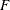and the vectors,, andin problem (NPSC).

Note: Althoughis a node-arc incidence matrix, it is specified in the ARCDATA= data set by arc definitions. Do not explicitly specify these flow conservation constraints as constraints of the problem.

In addition, the ARCDATA= data set can be used to specify information about nonarc variables, including objective function coefficients, lower and upper value bounds, and names. These data are the elements of the vectors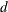,, andin problem ( NPSC). Data for an arc or nonarc variable can be given in more than one observation.

Supply and demand data also can be specified in the ARCDATA= data set. In such a case, the NODEDATA= data set may not be needed.

The CONDATA= data set describes the side constraints and their right-hand sides. These data are elements of the matrices
and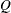and the vector. Constraint types are also specified in the CONDATA= data set. You can include in this data set upper bound values or capacities, lower flow or value bounds, and costs or objective function coefficients. It is possible to give all information about some or all nonarc variables in the CONDATA= data set.

An arc or nonarc variable is identified in this data set by its name. If you specify an arc’s name in the ARCDATA= data set, then this name is used to associate data in the CONDATA= data set with that arc. Each arc also has a default name that is the name of the tail and head node of the arc concatenated together and separated by an underscore character; tail_head, for example.

If you use the dense side constraint input format and want to use the default arc names, these arc names are names of SAS variables in the VAR list of the CONDATA= data set.

If you use the sparse side constraint input format (also described later) and want to use the default arc names, these arc names are values of the COLUMN list SAS variable of the CONDATA= data set.

When using the interior point algorithm, the execution of PROC NETFLOW has two stages. In the preliminary (zeroth) stage, the data are read from the NODEDATA= data set, the ARCDATA= data set, and the CONDATA= data set. Error checking is performed. The model is converted into an equivalent linear program.

In the next stage, the linear program is preprocessed. This is optional but highly recommended. Preprocessing analyzes the model and tries to determine before optimization whether variables can be fixed to their optimal values. Knowing that, the model can be modified and these variables dropped out. It can be determined that some constraints are redundant. Sometimes, preprocessing succeeds in reducing the size of the problem, thereby making the subsequent optimization easier and faster.

The optimal solution to the linear program is then found. The linear program is converted back to the original constrained network problem, and the optimum for this is derived from the optimum of the equivalent linear program. If the problem was preprocessed, the model is now post-processed, where fixed variables are reintroduced. The solution can be saved in the CONOUT= data set. This data set is also named in the PROC NETFLOW, RESET, and SAVE statements.

The interior point algorithm cannot efficiently be warm started, so options such as FUTURE1 and FUTURE2 options are irrelevant.

#### Introductory Example: Network Models: Interior Point Algorithm

Consider the following transshipment problem for an oil company in the section Introductory Example. Recall that crude oil is shipped to refineries where it is processed into gasoline and diesel fuel. The gasoline and diesel fuel are then distributed to service stations. At each stage there are shipping, processing, and distribution costs. Also, there are lower flow bounds and capacities. In addition, there are side constraints to model crude mix stipulations, and model the limitations on the amount of Middle Eastern crude that can be processed by each refinery and the conversion proportions of crude to gasoline and diesel fuel. The network diagram is reproduced in Figure 6.16.

Figure 6.16: Oil Industry Example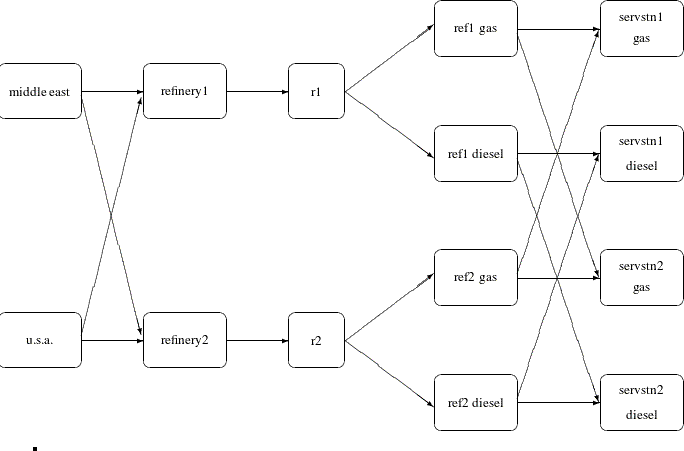To solve this problem with PROC NETFLOW, a representation of the model is saved in three SAS data sets that are identical to the data sets supplied to PROC NETFLOW when the simplex algorithm was used.

To find the minimum cost flow through the network that satisfies the supplies, demands, and side constraints, invoke PROC NETFLOW as follows:

```proc netflow
intpoint              /* <<<--- Interior Point used */
nodedata=noded        /* the supply and demand data */
arcdata=arcd1         /* the arc descriptions       */
condata=cond1         /* the side constraints       */
conout=solution;      /* the solution data set      */
run;
```

The following messages, which appear on the SAS log, summarize the model as read by PROC NETFLOW and note the progress toward a solution:

 Oil Industry Example Crude Oil can come from anywhere

 NOTE: Number of nodes= 14 . NOTE: Number of supply nodes= 2 . NOTE: Number of demand nodes= 4 . NOTE: Total supply= 180 , total demand= 180 . NOTE: Number of arcs= 18 . NOTE: Number of <= side constraints= 0 . NOTE: Number of == side constraints= 2 . NOTE: Number of >= side constraints= 2 . NOTE: Number of side constraint coefficients= 8 . NOTE: The following messages relate to the equivalent Linear Programming problem solved by the Interior Point algorithm. NOTE: Number of <= constraints= 0 . NOTE: Number of == constraints= 16 . NOTE: Number of >= constraints= 2 . NOTE: Number of constraint coefficients= 44 . NOTE: Number of variables= 18 . NOTE: After preprocessing, number of <= constraints= 0. NOTE: After preprocessing, number of == constraints= 3. NOTE: After preprocessing, number of >= constraints= 2. NOTE: The preprocessor eliminated 13 constraints from the problem. NOTE: The preprocessor eliminated 33 constraint coefficients from the problem. NOTE: After preprocessing, number of variables= 5. NOTE: The preprocessor eliminated 13 variables from the problem. NOTE: 4 columns, 0 rows and 4 coefficients were added to the problem to handle unrestricted variables, variables that are split, and constraint slack or surplus variables. NOTE: There are 10 sub-diagonal nonzeroes in the unfactored A Atranspose matrix. NOTE: The 5 factor nodes make up 1 supernodes NOTE: There are 0 nonzero sub-rows or sub-columns outside the supernodal triangular regions along the factors leading diagonal. NOTE: Bound feasibility attained by iteration 1. NOTE: Dual feasibility attained by iteration 1. NOTE: Constraint feasibility attained by iteration 1. NOTE: The Primal-Dual Predictor-Corrector Interior Point algorithm performed 6 iterations. NOTE: Optimum reached. NOTE: Objective= 50875. NOTE: The data set WORK.SOLUTION has 18 observations and 10 variables.

The first set of messages provide statistics on the size of the equivalent linear programming problem. The number of variables may not equal the number of arcs if the problem has nonarc variables. This example has none. To convert a network to an equivalent LP problem, a flow conservation constraint must be created for each node (including an excess or bypass node, if required). This explains why the number of equality side constraints and the number of constraint coefficients change when the interior point algorithm is used.

If the preprocessor was successful in decreasing the problem size, some messages will report how well it did. In this example, the model size was cut in half!

The following set of messages describe aspects of the interior point algorithm. Of particular interest are those concerned with the Cholesky factorization of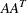where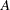is the coefficient matrix of the final LP. It is crucial to preorder the rows and columns of this matrix to prevent fill-in and reduce the number of row operations to undertake the factorization. See the section Interior Point Algorithmic Details for more explanation.

Unlike PROC LP, which displays the solution and other information as output, PROC NETFLOW saves the optimum in output SAS data sets you specify. For this example, the solution is saved in the SOLUTION data set. It can be displayed with PROC PRINT as

```
proc print data=solution;
var _from_ _to_ _cost_ _capac_ _lo_ _name_
_supply_ _demand_ _flow_ _fcost_ ;
sum _fcost_;
title3 'Constrained Optimum';
run;
```

Figure 6.17: CONOUT=SOLUTION

 Oil Industry Example Constrained Optimum

Obs _from_ _to_ _cost_ _capac_ _lo_ _name_ _SUPPLY_ _DEMAND_ _FLOW_ _FCOST_
1 refinery 1 r1 200 175 50 thruput1 . . 145.000 29000.00
2 refinery 2 r2 220 100 35 thruput2 . . 35.000 7700.00
3 r1 ref1 diesel 0 75 0   . . 36.250 0.00
4 r1 ref1 gas 0 140 0 r1_gas . . 108.750 0.00
5 r2 ref2 diesel 0 75 0   . . 8.750 0.00
6 r2 ref2 gas 0 100 0 r2_gas . . 26.250 0.00
7 middle east refinery 1 63 95 20 m_e_ref1 100 . 80.000 5040.00
8 u.s.a. refinery 1 55 99999999 0   80 . 65.000 3575.00
9 middle east refinery 2 81 80 10 m_e_ref2 100 . 20.000 1620.00
10 u.s.a. refinery 2 49 99999999 0   80 . 15.000 735.00
11 ref1 diesel servstn1 diesel 18 99999999 0   . 30 30.000 540.00
12 ref2 diesel servstn1 diesel 36 99999999 0   . 30 0.000 0.00
13 ref1 gas servstn1 gas 15 70 0   . 95 68.750 1031.25
14 ref2 gas servstn1 gas 17 35 5   . 95 26.250 446.25
15 ref1 diesel servstn2 diesel 17 99999999 0   . 15 6.250 106.25
16 ref2 diesel servstn2 diesel 23 99999999 0   . 15 8.750 201.25
17 ref1 gas servstn2 gas 22 60 0   . 40 40.000 880.00
18 ref2 gas servstn2 gas 31 99999999 0   . 40 0.000 0.00
50875.00

Notice that, in the `solution` data set (Figure 6.17), the optimal flow through each arc in the network is given in the variable named `_FLOW_`, and the cost of flow through each arc is given in the variable `_FCOST_`. As expected, the minimal total cost of the solution found by the interior point algorithm is equal to the minimal total cost of the solution found by the simplex algorithm. In this example, the solutions are the same (within several significant digits), but sometimes the solutions can be different.

Figure 6.18: Oil Industry Solution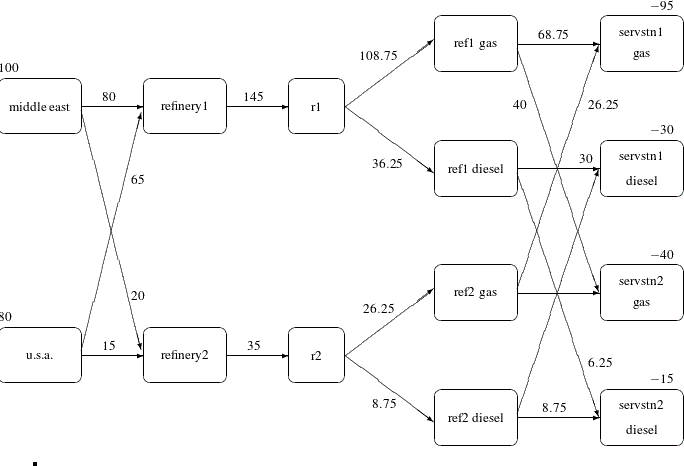#### Interactivity: Network Models: Interior Point Algorithm

PROC NETFLOW can be used interactively. You begin by giving the PROC NETFLOW statement with INTPOINT specified, and you must specify the ARCDATA= data set. The CONDATA= data set must also be specified if the problem has side constraints. If necessary, specify the NODEDATA= data set.

The variable lists should be given next. If you have variables in the input data sets that have special names (for example, a variable in the ARCDATA= data set named `_TAIL_` that has tail nodes of arcs as values), it may not be necessary to have many or any variable lists.

So far, this is the same as when the simplex algorithm is used, except the INTPOINT option is specified in the PROC NETFLOW statement. The PRINT, QUIT, SAVE, SHOW, RESET, and RUN statements follow and can be listed in any order. The QUIT statements can be used only once. The others can be used as many times as needed.

The CONOPT and PIVOT statements are not relevant to the interior point algorithm and should not be used.

Use the RESET or SAVE statement to change the name of the output data set. There is only one output data set, the CONOUT= data set. With the RESET statement, you can also indicate the reasons why optimization should stop (for example, you can indicate the maximum number of iterations that can be performed). PROC NETFLOW then has a chance to either execute the next statement, or, if the next statement is one that PROC NETFLOW does not recognize (the next PROC or DATA step in the SAS session), do any allowed optimization and finish. If no new statement has been submitted, you are prompted for one. Some options of the RESET statement enable you to control aspects of the interior point algorithm. Specifying certain values for these options can reduce the time it takes to solve a problem. Note that any of the RESET options can be specified in the PROC NETFLOW statement.

The RUN statement starts optimization. Once the optimization has started, it runs until the optimum is reached. The RUN statement should be specified at most once.

The QUIT statement immediately stops PROC NETFLOW. The SAVE statement has options that enable you to name the output data set; information about the current solution is put in this output data set. Use the SHOW statement if you want to examine the values of options of other statements. Information about the amount of optimization that has been done and the STATUS of the current solution can also be displayed using the SHOW statement.

The PRINT statement makes PROC NETFLOW display parts of the problem. The way the PRINT statements are specified are identical whether the interior point algorithm or the simplex algorithm is used, however there are minor differences in what is displayed for each arc, nonarc variable or constraint coefficient.

PRINT ARCS produces information on all arcs. PRINT SOME_ARCS limits this output to a subset of arcs. There are similar PRINT statements for nonarc variables and constraints:

```  PRINT NONARCS;
PRINT SOME_NONARCS;
PRINT CONSTRAINTS;
PRINT SOME_CONS;
```

PRINT CON_ARCS enables you to limit constraint information that is obtained to members of a set of arcs and that have nonzero constraint coefficients in a set of constraints. PRINT CON_NONARCS is the corresponding statement for nonarc variables.

For example, an interactive PROC NETFLOW run might look something like this:

```  proc netflow
intpoint      /* use the Interior Point algorithm */
arcdata=data set
other options;
variable list specifications;  /* if necessary        */
reset options;
print options;                 /* look at problem     */
run;                              /* do the optimization */
print options;    /* look at the optimal solution     */
save options;     /* keep optimal solution            */
```

If you are interested only in finding the optimal solution, have used SAS variables that have special names in the input data sets, and want to use default settings for everything, then the following statement is all you need.

`proc netflow intpoint arcdata=` data set ;

#### Functional Summary: Network Models, Interior Point Algorithm

The following table outlines the options available for the NETFLOW procedure when the interior point algorithm is being used, classified by function. An alphabetical list of options is provided in Dictionary of Options.

Table 6.7: Functional Summary, Network Models

Description

Statement

Option

Input Data Set Options:

Arcs input data set

Nodes input data set

Constraint input data set

Output Data Set Option:

Constrained solution data set

CONDATA has sparse data format

Default constraint type

Special COLUMN variable value

Special COLUMN variable value

Used to interpret arc and nonarc variable names

No new nonarc variables

No nonarc data in ARCDATA

Data for an arc found once in ARCDATA

Data for a constraint found once in CONDATA

Data for a coefficient found once in CONDATA

Data are grouped, exploited during data read

Problem Size Specification Options:

Approximate number of nodes

Approximate number of arcs

Approximate number of nonarc variables

Approximate number of coefficients

Approximate number of constraints

Network Options:

Default arc cost

Default arc capacity

Default arc lower flow bound

Network’s only supply node

SOURCE’s supply capability

Network’s only demand node

SINK’s demand

Convey excess supply/demand through network

Find maximal flow between SOURCE and SINK

Cost of bypass arc for MAXFLOW problem

Find shortest path from SOURCE to SINK

Memory Control Options:

Issue memory usage messages to SAS log

Number of bytes to use for main memory

Proportion of memory for arrays

Maximum bytes for a single array

Interior Point Algorithm Options:

Use interior point algorithm

Factorization method

Allowed amount of dual infeasibility

Allowed amount of primal infeasibility

Allowed total amount of dual infeasibility

Allowed total amount of primal infeasibility

Cut-off tolerance for Cholesky factorization

Density threshold for Cholesky processing

Step-length multiplier

Preprocessing type

Print optimization progress on SAS log

Write optimization time to SAS log

Interior Point Stopping Criteria Options:

Maximum number of interior point iterations

Primal-dual (duality) gap tolerance

Stop because of complementarity

Stop because of duality gap

Stop because of complementarity

Stop because of duality gap

Stop because of complementarity

Stop because of duality gap

Stop because of complementarity

Stop because of duality gap

PRINT Statement Options:

Display everything

Display arc information

Display nonarc variable information

Display variable information

Display constraint information

Display information for some arcs

Display information for some nonarc variables

Display information for some variables

Display information for some constraints

Display information for some constraints associated with some arcs

Display information for some constraints associated with some nonarc variables

Display information for some constraints associated with some variables

PRINT Statement Qualifiers:

Produce a short report

Produce a long report

Display arcs/variables with zero flow/value

Display arcs/variables with nonzero flow/value

SHOW Statement Options:

Show problem, optimization status

Show network model parameters

NETSTMT

Show data sets that have been or will be created

Miscellaneous Options:

Infinity value

Scale constraint row, nonarc variable column coefficients, or both